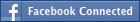• NEW! FREE Beat The GMAT QuizzesHundreds of Questions Highly Detailed Reporting Expert Explanations
• 7 CATs FREE!
If you earn 100 Forum Points

Engage in the Beat The GMAT forums to earn
100 points for $49 worth of Veritas practice GMATs FREEVERITAS PRACTICE GMAT EXAMS Earn 10 Points Per Post Earn 10 Points Per Thanks Earn 10 Points Per Upvote ## Geometry Problem ##### This topic has 1 expert reply and 3 member replies ABCD is a circle and circles are drawn with AO, CO, DO and OB as diameters. Areas E and F are shaded. E/F is equal to a. 1 b. 1/2 c. 1/π d. π/4 e. π/2### GMAT/MBA Expert GMAT InstructorJoined 21 Jan 2009 Posted: 3650 messages Followed by: 82 members Upvotes: 267 GMAT Score: 760vikrantr93 wrote: ABCD is a circle and circles are drawn with AO, CO, DO and OB as diameters. Areas E and F are shaded. E/F is equal to a. 1 b. 1/2 c. 1/π d. π/4 e. π/2Good question! Things to remember here are the areas of sectors and segments of a circle. Let’s take AD = BC = 2 r so that OA = OB = OC = OD = r and hence each included common chord (not named) is r √2/2. Area of each of the 8 segments = area of sector - area of triangle = ¼ X π X r^2 - ½ X r √2/2 X r √2/2 = ¼ X r^2 (π - 1) Hence total shaded F = 8 X ¼ X r^2 (π - 1) = 2 r^2 (π - 1). Area of big circle = π X (2 r) ^2 = 4 π r^2 Area inside the big circle EXCEPT the shaded E = area of 4 small circles - total shaded area F = 4 X π r^2 - 2 r^2 (π - 1) = 2 π r^2 + 2 r^2 = 2 r^2 (π + 1) Hence, total shaded area E = 4 π r^2 - (2 π r^2 + 2 r^2) = 2 π r^2 - 2 r^2 = 2 r^2 (π - 1). Hence E/F is equal to [2 r^2 (π - 1)]/ [2 r^2 (π - 1)] = 1 A _________________ The mind is everything. What you think you become. –Lord Buddha Sanjeev K Saxena Quantitative Instructor The Princeton Review - Manya Abroad Lucknow-226001 www.manyagroup.com Free GMAT Practice Test How can you improve your test score if you don't know your baseline score? Take a free online practice exam. Get started on achieving your dream score today! Sign up now. Legendary MemberJoined 17 May 2011 Posted: 1448 messages Followed by: 53 members Upvotes: 375 vikrantr93 wrote: ABCD is a circle and circles are drawn with AO, CO, DO and OB as diameters. Areas E and F are shaded. E/F is equal to a. 1 b. 1/2 c. 1/π d. π/4 e. π/2Hi, I dont know if some of the unshaded regions marked as F are mistyped as G. The question states E and F are shaded regions. so, I am taking for granted that the 4 inner shaded regions are F and the four outer shaded regions are E Let the radius of smaller circles be r. So, the radius of outer circle is 2r Adding the the areas of 4 smaller circles gives 4.πr^2 = unshaded region + 2.F (as each shaded F is repeated twice) So, 4.πr^2 = unshaded region + 2F --eqn(1) The area of outer circle is π(2r)^2 = 4πr^2 = unshaded region+F+E --eqn(2) From eqns(1) & (@) we get 4.πr^2 = unshaded region + 2F = unshaded region+F+E => E = F. So, E/F = 1 Hence, A Cheers! Legendary MemberJoined 04 Apr 2011 Posted: 1309 messages Followed by: 123 members Upvotes: 310 Test Date: 13th Oct Target GMAT Score: 750+ GMAT Score: 750Isn't there a problem with the question?? Legendary MemberJoined 17 May 2011 Posted: 1448 messages Followed by: 53 members Upvotes: 375 cans wrote: Isn't there a problem with the question?? Hi, I think some of the unshaded regions are mistakenly marked 'F' instead of 'G'. But, in the ques, it is mentioned that E and F are marked regions. So, I have assumed that in my post. Moreover, the options seem to be having 'n'. In fact, it is π(Pie). Cheers! •Free Practice Test & Review How would you score if you took the GMAT Available with Beat the GMAT members only code •1 Hour Free BEAT THE GMAT EXCLUSIVE Available with Beat the GMAT members only code •Award-winning private GMAT tutoring Register now and save up to$200

Available with Beat the GMAT members only code

•5 Day FREE Trial
Study Smarter, Not Harder

Available with Beat the GMAT members only code

•Free Veritas GMAT Class
Experience Lesson 1 Live Free

Available with Beat the GMAT members only code

•5-Day Free Trial
5-day free, full-access trial TTP Quant

Available with Beat the GMAT members only code

•Magoosh
Study with Magoosh GMAT prep

Available with Beat the GMAT members only code

•FREE GMAT Exam
Know how you'd score today for \$0

Available with Beat the GMAT members only code

•Free Trial & Practice Exam
BEAT THE GMAT EXCLUSIVE

Available with Beat the GMAT members only code

•Get 300+ Practice Questions

Available with Beat the GMAT members only code

### Top First Responders*

1Ian Stewart 44 first replies
2Brent@GMATPrepNow 32 first replies
3Scott@TargetTestPrep 31 first replies
4Jay@ManhattanReview 31 first replies
5GMATGuruNY 18 first replies
* Only counts replies to topics started in last 30 days
See More Top Beat The GMAT Members

### Most Active Experts

1Scott@TargetTestPrep

Target Test Prep

138 posts
2Max@Math Revolution

Math Revolution

85 posts
3Ian Stewart

GMATiX Teacher

53 posts
4Brent@GMATPrepNow

GMAT Prep Now Teacher

50 posts
5Jay@ManhattanReview

Manhattan Review

31 posts
See More Top Beat The GMAT Experts